SSC  >  Quantitative Aptitude - Test 2

# Quantitative Aptitude - Test 2

Test Description

## 25 Questions MCQ Test SSC CGL Tier 1 Mock Test Series | Quantitative Aptitude - Test 2

Quantitative Aptitude - Test 2 for SSC 2022 is part of SSC CGL Tier 1 Mock Test Series preparation. The Quantitative Aptitude - Test 2 questions and answers have been prepared according to the SSC exam syllabus.The Quantitative Aptitude - Test 2 MCQs are made for SSC 2022 Exam. Find important definitions, questions, notes, meanings, examples, exercises, MCQs and online tests for Quantitative Aptitude - Test 2 below.
Solutions of Quantitative Aptitude - Test 2 questions in English are available as part of our SSC CGL Tier 1 Mock Test Series for SSC & Quantitative Aptitude - Test 2 solutions in Hindi for SSC CGL Tier 1 Mock Test Series course. Download more important topics, notes, lectures and mock test series for SSC Exam by signing up for free. Attempt Quantitative Aptitude - Test 2 | 25 questions in 15 minutes | Mock test for SSC preparation | Free important questions MCQ to study SSC CGL Tier 1 Mock Test Series for SSC Exam | Download free PDF with solutions
 1 Crore+ students have signed up on EduRev. Have you?
Quantitative Aptitude - Test 2 - Question 1

### Find the approximate area of a trapezium whose parallel sides are 22 cm, 12 cm and the other sides are 8 cm and 6 cm.

Detailed Solution for Quantitative Aptitude - Test 2 - Question 1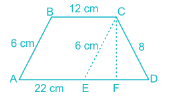Consider the trapezium ABCD,
where AB = 6 cm, BC = 12 cm, CD = 8 cm and AD = 22 cm.
Draw a line from C parallel to AB at E such that ABCE is a parallelogram.
∴ CE = AB = 6 cm and AE = BC = 12 cm.
⇒ ED = AD – AE
⇒ ED = 22 – 12 = 10 cm.
Area of Trapezium ABCD = Area of Parallelogram ABCE + Area of ΔCED
Draw a line through C to ED such that CF is perpendicular to ED.
Area of ΔCED:
From Heron’s formulae,
Δ CED = √[(s) (s - a) (s - b) (s - c)], where s = (a + b + c)/2 and a, b, c are side of the triangle.
In this case, a = 6; b = 8 and c = 10
⇒ s = 24/2 = 12
Area of ΔCED = √(12 × 6 × 4 × 2)
⇒ Area of ΔCED = √(576) = 24 sq. cm.
Area of ΔCED = (1/2) × ED × CF
⇒ 24 = (1/2) × 10 × CF
⇒ CF = 4.8 cm.
Area of Parallelogram ABCE = AE × CF
⇒ Area of Parallelogram ABCE = 12 × 4.8 = 57.6 sq. cm.
Area of trapezium ABCD = Area of parallelogram ABCE + Area of ΔCED
⇒ Area of trapezium ABCD = 57.6 + 24 = 81.6 sq. cm.
∴ Approximate area of the trapezium = 81.6 sq. cm.

Quantitative Aptitude - Test 2 - Question 2

### A, B and C enter into a partnership, investing Rs. 6000. A invests Rs. 1000 and B and C invests in the ratio of 2 : 3. Find the profit of C, when the annual profit is Rs. 2400

Detailed Solution for Quantitative Aptitude - Test 2 - Question 2

From given data,
Total investment = Rs. 6000
A investment = Rs. 1000
⇒ B and C’s investment = 6000 – 1000 = Rs. 5000
The ratio of B and C’s investment = 2 : 3
⇒ B’s investment = 2/5 × 5000 = Rs. 2000
⇒ C’s investment = 3/5 × 5000 = Rs. 3000
∴ ratio of their investment = 1000 : 2000 : 3000 = 1 : 2 : 3
Given that the annual profit = Rs. 2400
Let their profits be x : 2x : 3x
⇒ 2400 = x + 2x + 3x
⇒ 6x = 2400
⇒ x = Rs. 400
∴ C’s profit = 3x = 3 × 400 = Rs. 1200

Quantitative Aptitude - Test 2 - Question 3

### A train 150 metres long crosses a pole in 15 seconds and crosses another train of the same length travelling in the opposite direction in 12 seconds. The speed of the second train in km/hr is

Detailed Solution for Quantitative Aptitude - Test 2 - Question 3

A train 150 metres long crosses a pole in 15 seconds
Distance covered to cross the pole = Length of the train = 150 m
Time required = 15 sec
∴ Speed of the first train
= Distance covered/Required time
= 150m/15sec = 10 m/sec
Let, Speed of the second train = ‘x’ m/sec
∵ Trains were travelling in opposite directions
∴ Relative velocity of first train with respect to second train
= (10 + x) km/sec
Given,
First train takes 12 second to cross the second train moving in opposite direction
∴ Distance covered to cross second train
= Length of first train + Length of second train
∵ Length of second train = Length of first train
= 150 m + 150 m = 300 m
And, Time required = 12 sec
∴ Speed of the first train = Distance covered/Required time
⇒ 10 + x = 300/12
⇒ 120 + 12x = 300
⇒ 12x = 180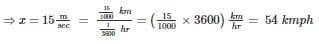Quantitative Aptitude - Test 2 - Question 4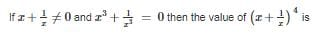Detailed Solution for Quantitative Aptitude - Test 2 - Question 4

From the given data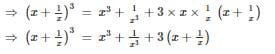But we know that x3 + 1/x3 = 0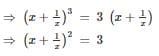Squaring on both sides, we get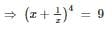Quantitative Aptitude - Test 2 - Question 5

Trish and Stacy can complete a work in 20 days and 30 days, respectively, when working alone. In an arrangement, Trish works for 2 days, and then Stacy works for a day. They keep on repeating this process till the work gets completed. On which day will the work gets completed?​

Detailed Solution for Quantitative Aptitude - Test 2 - Question 5

In given arrangement, Trish works for 2 days, and then Stacy works for a day. This cycle repeats till work is completed.
Fraction of work done by Trish and Stacy in a day is 1/20 and 1/30, respectively.
Work completed in 1 cycle of 3 days = 2/20 + 1/30 = 4/30
⇒ Work completed in 7 cycles of 3 days = 28/30
Work done till first day of 8th cycle = 28/30 + 1/20 = 59/60
Work done till second day of 8th cycle = 59/60 + 1/20 = 62/60
⇒ Work gets completed on 2nd day of 8th cycle, i.e., on 23rd day.

Quantitative Aptitude - Test 2 - Question 6

If the sum of the measures of all the interior angles of polygon is 1080°, find the number of sides of the polygon?

Detailed Solution for Quantitative Aptitude - Test 2 - Question 6

We know that sum of the interior angles of a polygon of n sides = 180 (n - 2)
Given that sum of the measures of all the interior angles = 1080°
⇒ 180(n – 2) = 1080
⇒ n – 2 = 6
⇒ n = 8

Quantitative Aptitude - Test 2 - Question 7

If 2cosec2A = x, then find the value of x?

Detailed Solution for Quantitative Aptitude - Test 2 - Question 7

From given data, we get
⇒ 2cosec2 A = x
⇒ 2/sin2 A = x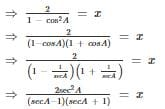Quantitative Aptitude - Test 2 - Question 8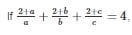then the value of (ab + bc + ca)/abc is

Detailed Solution for Quantitative Aptitude - Test 2 - Question 8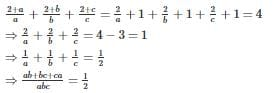Quantitative Aptitude - Test 2 - Question 9

In an examination, 35% of total students failed in Hindi, 45% failed in English and 20% failed in both. Find the percentage of those students who passed in both the subjects?

Detailed Solution for Quantitative Aptitude - Test 2 - Question 9

From given data
No of students failed in Hindi n(H) = 35%
No of students failed in English n(E) = 45%
No of students failed in both n(H ∩ E) = 20%
⇒ total number of students who failed n(H ⋃ E) = n(H) + n(E) – n(H ∩ E)
⇒ n(H ⋃ E) = 35 + 45 - 20
⇒ n(H ⋃ E) = 60
No of students who passed = 100 - (total no of students who failed) = 100 – 60 = 40%

Quantitative Aptitude - Test 2 - Question 10

A sum of Rs. 3000 amounts to Rs. 6000 in two years at compound interest. The interest for four years is

Detailed Solution for Quantitative Aptitude - Test 2 - Question 10

From the given data
We know amount = p(1 + r/100)n
⇒ 3000(1 + r/100)2 = 6000
⇒ (1 + r/100)2 = 2
Compound interest for four years = amount – principle
= p(1 + r/100)n – principle
= 3000(1 + r/100)– 3000 = 12000 – 3000 = Rs. 9000

Quantitative Aptitude - Test 2 - Question 11

If the diagonals of a rhombus are 24 and 10, then the value of thrice its side is

Detailed Solution for Quantitative Aptitude - Test 2 - Question 11

Half of each of the two diagonal forms the two sides of a right - angled triangle whose hypotenuse is the side of the rhombus.
Length of Diagonal 1 = 24
Length of Diagonal 2 = 10
⇒ Sides of the triangle = 12 and 5

Since it’s a right - angled triangle, it should follow Pythagoras theorem.
⇒ (Side)2 = (12)2 + (5)2
⇒ Side = 13
∴ Side of the rhombus = 13.
⇒ Thrice the value of the side = 3 × 13 = 39

Quantitative Aptitude - Test 2 - Question 12

If the measures of the angles of a triangle are in the ratio. 1 ∶ 2 ∶ 3 and if the length of the smallest side of the triangle is 10 cm, then the length of the longest side is

Detailed Solution for Quantitative Aptitude - Test 2 - Question 12

Let the angles of the triangles are in the ratio x ∶ 2x ∶ 3x
We know that sum of the angles in a triangle = 180°
⇒ x + 2x + 3x = 180
⇒ 6x = 180°
⇒ x = 30°
by sin formula sinA/a = sinB/b = sinC/c
∴ the angles of the triangle are 30°, 60° and 90°, so the sides are in the ratio of 1 ∶ 2 ∶ √3
∴ smallest side will be opposite to 30°
Let the sides of the triangle are in the ratio of x : 2x : √3x
But given smallest side x = 10 cm
⇒ remaining sides are 2x = 2(10) = 20 cm and √3x = √3(10) = 17.32 cm
∴ the longest side = 20 cm

Quantitative Aptitude - Test 2 - Question 13

Two numbers are respectively 30% and 80% more than a third number. Find the ratio of these numbers.

Detailed Solution for Quantitative Aptitude - Test 2 - Question 13

Let the third number be X.
Let the first number be A, and the other number be B. We have,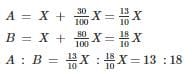Quantitative Aptitude - Test 2 - Question 14

A cricketer has a certain average of runs for his 6 innings. In the seventh inning he scores 100 runs, thereby increasing his average by 7 runs. His new average of runs is

Detailed Solution for Quantitative Aptitude - Test 2 - Question 14

Let the average of 6 innings be x.
Then new average can be found by (6x + 100)/7 = x + 7
⇒ 6x + 100 = 7x + 49
⇒ x = 51
∴ New average = 51 + 7 = 58

Quantitative Aptitude - Test 2 - Question 15

If a3 ­ - b3 = 56 and a ­ - b = 2 then what is the value of a2 + b2?​

Detailed Solution for Quantitative Aptitude - Test 2 - Question 15

a– b3 = (a – b)(a2 + ab + b2)
56 = 2(a2 + ab + b2)
28 = (a2 + ab + b2) ----(1)
Now, (a – b)2 = a2 – 2ab + b2 = 4 ----(2)
Solving equation (1) and (2),
a2 + ab + b2 = 28
a2 – 2ab + b2 = 4
3ab = 24
ab = 8
Therefore, a2 + 8 + b2 = 28 in (1)
And a2 + b2 = 28 – 8 = 20

Quantitative Aptitude - Test 2 - Question 16

In ΔABC, ∠B = 5∠C and ∠A = 3∠C, then the measure of ∠C is

Detailed Solution for Quantitative Aptitude - Test 2 - Question 16

We know that in a triangle,
Sum of angles = 180°
⇒ ∠A + ∠B + ∠C = 180°
⇒ 3∠C + 5∠C + ∠C = 180°
⇒ 9∠C = 180°
⇒ ∠C = 180/9 = 20°
∴ ∠C = 20°

Quantitative Aptitude - Test 2 - Question 17

A pole stands vertically, inside a scalene triangular park ABC. If the angle of elevation of top of the pole from each corner of the park is same, then in Δ ABC, the foot of the pole is at the

Detailed Solution for Quantitative Aptitude - Test 2 - Question 17

tanθ = perpendicular/base
Given, a pole stands vertically, inside a scalene triangular park ABC. The angle of elevation of top of the pole from each corner of the park is same.
If the angle of elevation is same then, the distance of each corner from the foot of the would also be same,
Base = perpendicular/tanθ
Perpendicular is height of the pole while θ is the angle of elevation.
For the above to be true, the foot of the pole should be a circumcenter and the distance of each corner from the foot is the circumradius.

Quantitative Aptitude - Test 2 - Question 18

Three sides of a triangle are 5 cm, 9 cm and x cm. The minimum integral value of x is

Detailed Solution for Quantitative Aptitude - Test 2 - Question 18

From the given data
Length of sides of a triangle are of 5 cm, 9 cm and x cm
⇒ length of the third side must be less than the sum of the other two sides
⇒ x < 5 + 9 or x < 14
We also know that length of the third side must be greater than difference of the other two sides
⇒ x > 9 – 5 or x > 4
⇒ By both the conditions 4 < x < 14

Quantitative Aptitude - Test 2 - Question 19

The length of a chord which is at a distance of 5cm from the centre of a circle of radius 13cm is

Detailed Solution for Quantitative Aptitude - Test 2 - Question 19

From given data
Let AB be a chord of circle with center O and radius OA and OB equals to 13 cm
Draw OP perpendicular to AB
Given that OP = 5 cm
From ΔOPA, we know that
⇒ OA2 = AP2 + OP2
⇒ 132 = AP2 + 52
⇒ 169 – 25 = AP2
⇒ AP = 12 cm
But we know that AB = 2 AP = 2× 12 = 24 cm

Quantitative Aptitude - Test 2 - Question 20

Divide 43 into two parts so that 4 times the first and 3 times the second together equals to 150. Then ratio of the first and second parts is

Detailed Solution for Quantitative Aptitude - Test 2 - Question 20

Let the first part of 43 be x, then the second part will be 43 – x
According to the question,
4x + 3 × (43 – x) = 150
⇒ 4x + 129 – 3 x=150
⇒ x = 21
∴ The first part is 21 and second part is 43 – 21 = 22

Quantitative Aptitude - Test 2 - Question 21

25% discount is already offered on an item. By applying a promo code the customer wins 20% cash back. What is the effective discount?

Detailed Solution for Quantitative Aptitude - Test 2 - Question 21

Let the cost price of the item be Rs. 100
25% discount is offered on an item
⇒ discount = 100 × 25/100 = Rs. 25
∴ discounted selling price = 100 - 25 = Rs. 75
Again 20% cash back is offered
⇒ cash back amount = 75 × 20/100 = Rs. 15
∴ final selling price = 75 - 15 = Rs. 60
Effective discount = cost price – selling price/cost price × 100 = 100 - 60/100 × 100 = 40%

Quantitative Aptitude - Test 2 - Question 22

Refer the below data table and answer the following Question :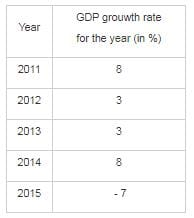If the GDP of the country was \$3 trillion at the end of 2013, what was it at the beginning of 2015?​

Detailed Solution for Quantitative Aptitude - Test 2 - Question 22

As per the given data,
3\$ was the GDP at the end of 2013.
It was given that to find at the beginning of 2015, it means to find the GDP at the end of 2014. At the end of 2014, there was the increase of GDP to 8%.
⇒ total amount at the end of 2014 = 3 trillion + (8% of 3 trillion) = 3.24 trillion
∴ GDP at the beginning of 2015 was \$3.24 trillion.

Quantitative Aptitude - Test 2 - Question 23

Refer the below data table and answer the following Question :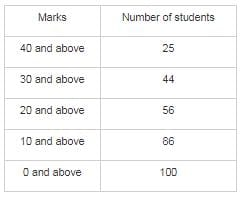Q. Find the number of students whose marks are between 30 and 40 ?

Detailed Solution for Quantitative Aptitude - Test 2 - Question 23

As per the given data
Similarly no of students who got marks 30 and above = 44
Similarly no of students who got marks 40 and above = 25
∴ no of students whose marks are between 30 and 40 = 44 – 25 = 19

Quantitative Aptitude - Test 2 - Question 24

Direction: Study the following graph and answer the question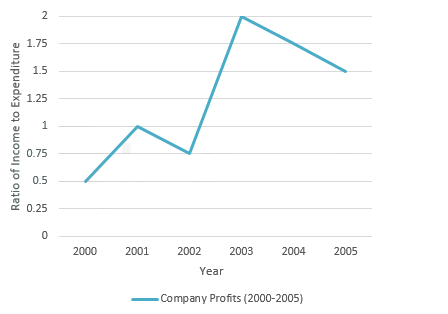Q. If the profit made in the year 2003 was Rs. 450000, then find the income

Detailed Solution for Quantitative Aptitude - Test 2 - Question 24

From the line graph,
Ratio of income to expenditure in the 2003 = 2
Let the expenditure be a, ∴ income = 2a
Profit = income – expenditure
⇒ 450000 = 2a – a
⇒ a = Rs. 450000
Thus, income = 2a = Rs. 900000

Quantitative Aptitude - Test 2 - Question 25

Direction: Study the following graph and answer the questionQ. Find the percentage increase in income from 2002 to 2003​

Detailed Solution for Quantitative Aptitude - Test 2 - Question 25

The line graph is drawn between ratio of income to expenditure and annual year.
We don’t know the income in each year.
Thus, the data given is inadequate.

## SSC CGL Tier 1 Mock Test Series

42 docs|103 tests
 Use Code STAYHOME200 and get INR 200 additional OFF Use Coupon Code
Information about Quantitative Aptitude - Test 2 Page
In this test you can find the Exam questions for Quantitative Aptitude - Test 2 solved & explained in the simplest way possible. Besides giving Questions and answers for Quantitative Aptitude - Test 2, EduRev gives you an ample number of Online tests for practice

## SSC CGL Tier 1 Mock Test Series

42 docs|103 tests